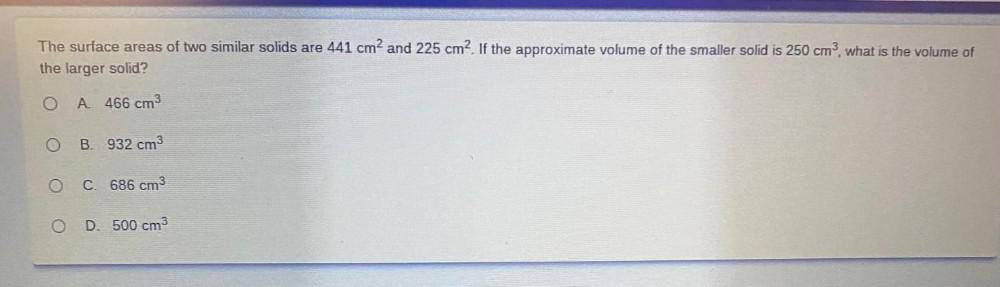Question:

# The surface areas of two similar solids are 441 cm2 and 225 cm2. If the approximate volume of the smaller solid is 250 cm?, whatThe surface areas of two similar solids are 441 cm2 and 225 cm2. If the approximate volume of the smaller solid is 250 cm?, what is the volume of the larger solid? O A 466 cm3 O B. 932 cm3 C. 686 cm3 O D. 500 cm3